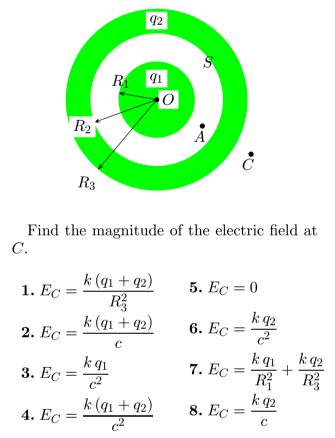Physics Practice Problems Gauss' Law Practice Problems Solution: A solid conducting sphere of radius R1 and total c...

# Solution: A solid conducting sphere of radius R1 and total charge q1 is enclosed by a conducting shell with an inner radius R2 and outer radius R3 and total charge q2. OA = a and OC = c.

###### Problem

A solid conducting sphere of radius R1 and total charge q1 is enclosed by a conducting shell with an inner radius R2 and outer radius R3 and total charge q2. OA = a and OC = c.Gauss' Law

Gauss' Law

#### Q. The electric field is constant over each face of the cube shown in the figure. Does the box contain positive charge, negative charge, or no charge?  ...

Solved • Mon Apr 10 2017 11:46:29 GMT-0400 (EDT)

Gauss' Law

#### Q. A cubic box of side a, oriented as shown, contains an unknown charge. The vertically directed electric field has a uniform magnitude E at the top surf...

Solved • Thu Jan 12 2017 15:30:35 GMT-0500 (EST)

Gauss' Law

#### Q. A charge is placed in a closed box. If the size of the box doubles, the electric flux going through the boxA) reverse the sign but keeps the same magn...

Solved • Thu Jan 05 2017 13:56:42 GMT-0500 (EST)

Gauss' Law

#### Q. A solid conducting sphere of radius R1 and total charge q1 is enclosed by a conducting shell with an inner radius R2 and outer radius R3 and total cha...

Solved • Wed Jan 04 2017 15:21:21 GMT-0500 (EST)